Wednesday, 29 August 2012

Force on Current-Carrying Conductor in a Magnetic Field

• Interaction of Magnetic Fields:
The Resultant Field with Catapult Effect (a.k.a. Catapult Field)
i.      Just as two bar magnets coming within the magnetic field of each other would experience a force – a push (repulsion) or a pull (attraction) depending on the positions of their magnetic poles:

i.      a current-carrying conductor (which has magnetic field around it) would similarly experience a magnetic force when its magnetic field interacts with the magnetic field of another magnet.

ii.      The interaction produces a resultant magnetic field (a.k.a. catapult field) with magnetic field lines wrapping around the conductor like the stretched catapult producing a catapult effect – that moves the conductor from stronger region of the magnetic field to weaker region.
iii.      Drawing the ‘catapult field’ is one way to determine the direction of movement of the current-carrying conductor in a magnetic field – however, it could be time-consuming. A much simpler method is by the use of Fleming’s Left-hand Rule (or Motor Rule):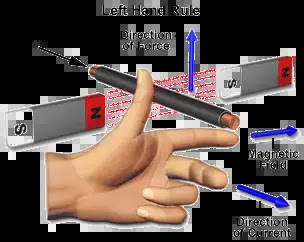Alternatively, the Right-hand Slap Rule where the four fingers of the right point in the direction of the magnetic field B and the thumb points in the direction of the current  I, the direction of slapping would be the direction of force F on the conductor.: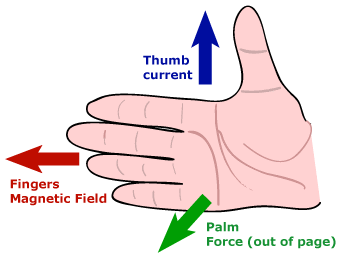iv.      Interestingly, the magnetic field of a current-carrying conductor itself would also exert a force on the other magnet which field exerts a force on it - as Hans Christian Oersted discovered in 1820 when the pointer of a compass moved when current was switched on in a nearby circuit – It is this discovery which led to the theory of electromagnetism and its many useful applications.

ii.      Fleming’s Left Hand Rule (Motor Rule)
Please see above for the diagram.

iii.      Factors Affecting Magnitude of the Force on Current-Carrying Conductor in a Magnetic Field:
i.      Magnetic Field Strength (of the other magnet)
ii.      Size of Current (in the conductor causing induced magnetic field)
iii.      Length of Wire or Conductor in the Field
iv.      Angle between Magnetic Field (B) and the Current (I)

iv.      Force between Two Current-Carrying Conductors:i.      The magnetic fields of two current-carrying conductors would interact with each other as would the fields of two permanent magnets.

ii.      If the two conductors are near (but not next) to each other:
i.      They attract each other when the current is flowing in the same direction in both of them;
ii.      They repel each other when the current is flowing in the opposite direction.
The direction of movement of the conductor can be determined by any of the following methods:
a.       Plotting the catapult field
b.      Fleming’s Left-hand Rule
c.       Right-hand Slap Rule

iii.      If the two conductors are next to each other:
i.      They interact to produce a stronger magnetic field when the current is flowing in the same direction in both of them;
ii.      They interact to produce a weaker or neutral field when the current is flowing in the opposite direction.

v.      Turning Effect of a Current-Carrying Coil in a Magnetic Field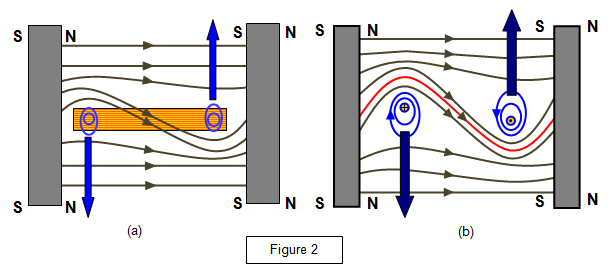i.      For a coil placed in a magnetic field, when the current flows through it, it flows in opposite directions in opposing arms of the coil – concentric magnetic field lines in opposite directions are therefore produced around both arms.

ii.      These induced magnetic fields interact with the external magnetic field. The resultant magnetic field produces a catapult effect in opposite directions for opposing arms of the coil – forming a couple which produces a turning effect and so the coil rotates.

iii.      The direction of rotation of the coil would reverses when:
i.      The current reverses
ii.      The external magnet poles reverse

The direction of rotation of the coil can be determined by:
1.      Plotting the catapult field; or,
2.      Fleming’s Left-hand Rule; or,
3.      Right-hand Slap Rule

vi.      D.C. Motor – Factors Affecting Speed of Rotation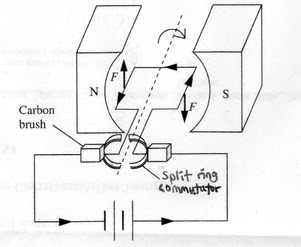i.      The diagram above shows the construction features of a simple dc motor.

ii.      A dc motor consists of a rectangular coil of wire placed between two permanent magnets (a.k.a. the external magnets).

iii.      Both ends of the coil are soldered to a commutator made up of two semi circular copper rings.

iv.      Two carbon brushes are held against the commutator with slight pressure with the help of springs.

v.      Because of the commutator, current always flow in the same direction for the arm near to the north-pole of the external magnet and in the opposite direction for the other arm of the coil. As a result, the coil rotates in one direction.

vi.      The speed of rotation of the coil can be increased if any of these is increased:
1.      The Magnetic Field Strength (of the external magnet)
2.      The Current flowing in the coil (causing stronger induced magnetic field)
3.      The Number of Turns in the Coil (causing stronger induced magnetic field)
4.      The Area of the Coil
------------------------------------------------------------

Tuesday, 21 August 2012

Magnetic Effect of Current-Carrying Conductor

(Reviewed and updated on 03/06/2016):

i.            Ferromagnetic Materials and Non-Magnetic Materials:

a.       Materials which are attracted to magnets are known as ferromagnetic materials or simply as ferrous or magnetic materials. These include, iron, cobalt and nickel as well as the iron-containing alloy, steel.

b.      Materials which are not attracted to magnets are known as non-magnetic materials. These include glass, wood and such metals as aluminium, zinc, lead, copper, silver, gold and alloy such as brass.

ii.            Magnetic Field (Definition, Causes, Direction and Poles):

a.       Definition: A magnetic field is a space within which a magnetic material and a magnet would experience a force.

b.      Causes: A magnetic field is created around:

i.      a magnet such as lodestone and  artificial magnet;

ii.      a current-carrying conductor (including electromagnet);

iii.  the rotating Earth.
------------------------------------------------------

i) Magnetic Field Lines of Bar Magnet:In the diagram, note that:
* the field lines have arrows on them
* the field lines come out of N and go into S
* the field lines are more concentrated at the poles.
The magnetic field is strongest at the poles, where the field lines are most concentrated.
---------------------------------------------------
ii)  A diagram of the magnetic field produced by a current-carrying wire is shown below:------------------------------------------------------------------------------

c.       Direction of Magnetic Lines of Force:

The direction of the magnetic lines of force at any point within a magnetic field is the direction:
i.      that a free north pole placed at that point would move; or,
ii.     that a plotting compass placed at that point would point.

This direction always begins at the north pole and ends at the south pole.

At the geographic north of our Earth, there appears to be a magnetic south pole; and conversely, at the geographic south, there appears to be a magnetic north pole - pl. see diagram below:--------------------------------------------------------------------------------

d.      Poles of Magnet:
i.      North pole of a magnet refers to that part of the magnet which would repel the north pole of another magnet or which would be attracted to the south pole of another magnet. When a bar magnet is freely suspended (as in a compass), the north pole (arrow-head) would point approximately to the geographic north of the Earth which contains a magnetic south pole.

ii.      South pole of a magnet refers to the pole which is opposite to and attracts the north pole of the magnet. When a bar magnet is freely suspended, the south pole would approximately point to the Earth’s geographic south which contain a magnetic north pole.

iii.      A magnet, depending on its shape, invariably has at least a pair of opposite poles.

iii.            Magnetic Field (pattern, direction, strength) in Current-Carrying Conductor:

i.      When there is current flowing in a conductor, a magnetic field is created in the space around the conductor. And, the conductor may be of any shape such as:
a.      a straight wire
b.      a coil; or,
c.       a solenoid (multiple continuous coils)

ii.      The pattern and direction of the magnetic field created due to current in a conductor depends on the shape of the conductor and the direction of current:

a.      In a Straight Wire

i.      The pattern of magnetic field lines is that of concentric circles around the conductor.
ii.      The direction of the magnetic field lines may be summarized by:
1.      Right-hand Grip Rule (diagram above); or,
2.      Maxwell’s Screw Rule (diagram below)
iii.      The strength of the magnetic field is:
1.      stronger near the current than further away
2.      stronger when the current is increased

iv.      when the direction of the current changes,
1.      the pattern remains the same
2.      the direction of the magnetic field lines reverses
3.      the strength of the magnetic field depends on the same two factors:
a.       proximity (nearness) to current; and,
b.      size of the current.

b.      In a Coil
Magnetic Field Around a Coili.      The pattern of magnetic field lines is that of compressed circles around the conductor with the circles flattened within the coil resulting in the lines more closely spaced within the coil than outside it and at the centre, the line is straight.

ii.      The direction of the magnetic field lines around the coil at any point may be summarized by

1.      Right-hand Grip Rule;
2.      Looking at the direction of the current from each end of the coil.

The field lines around the two wires are in opposite direction.

iii.      The strength of the magnetic field is:
1.      stronger within the coil than outside the coil; and,
2.      stronger when the current is increased

iv.      when the direction of the current reverses,

1.      the pattern remains the same;

2.      but the direction of the magnetic field lines around each wire reverses;

3.      the strength of the magnetic field depends on the same factors:
a.       stronger within the coil; and,
b.      stronger when the current increases.

c.      Magnetic Field Around a Solenoid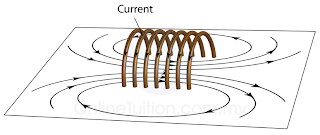i.      The pattern of magnetic field lines is that of compressed circles around the conductor with the circles flattened within the solenoid resulting in the lines more closely and evenly spaced within the coil than outside it and at the centre, the line is straight.

ii.      The direction of the magnetic field lines around the solenoid and the north pole and south pole of the solenoid may be summarized by

1.      Right-hand Grip Rule; or,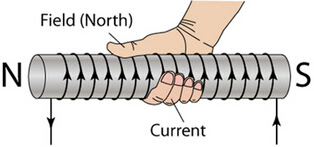2.      Looking at the direction of the current from each end of the solenoid.The direction of the field lines inside the solenoid is opposite to the direction of the field lines outside it.

iii.      The strength of the magnetic field is:

1.      stronger and more even within the solenoid than outside the coil; and,
2.      stronger when the current is increased

iv.      when the direction of the current reverses,
1.      the pattern remains the same;

2.      but the direction of the magnetic field lines around each wire reverses;

3.      the strength of the magnetic field depends on the same factors:
a.       stronger within the coil; and,
b.      stronger when the current increases.

v.   Experiment in Activity 3.3 at pg. 402 provides the above facts.

iii.      Factors Affecting Strength of Electromagnet

a.       An electromagnet is a temporary magnet consisting of soft ferromagnetic material (usually soft iron) surrounded by solenoid of many turns of insulated copper wire. When current flows through the solenoid, the soft ferromagnetic material becomes a magnet; when the current is switched off, the magnetism is lost.

b.      A simple electromagnet consists of a soft iron core surrounded by a solenoid.A simple electromagnet consists of a solenoid of many turns of insulated wire wrapped around an iron core. The strength of magnetic field generated is proportional to the amount of current as well as factors stated below.

c.       The strength of an electromagnet depends on:
i.      The size of the current flowing through the solenoid
ii.      The number of turns of the coils in the solenoid
iii.      The soft ferromagnetic material used – soft iron being ideal
iv.      The shape of the soft ferromagnetic material used – M-shaped being better than U-shaped; and U-shaped being better than a cylindrical core;

iv.      Uses of Electromagnets
a.       Electromagnetic Lifting Machineb.      The Electric Bell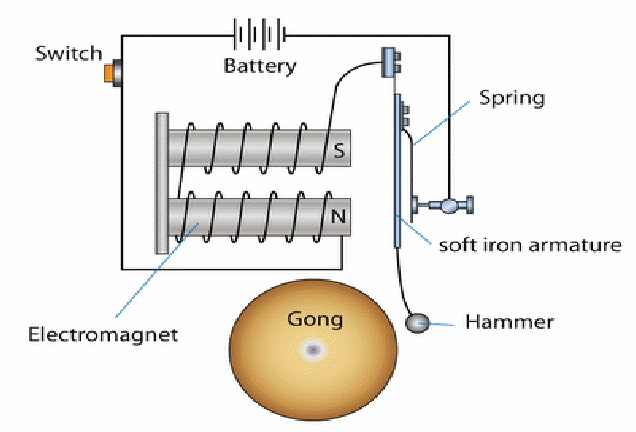c.       The Electromagnetic Relayd.      The Telephone Earpiece

How does a telephone earpiece work?

e.       The Circuit Breaker

The Working Of A Circuit Breakers Through A Diagram :